# Overview of Forces & Gravity Flashcards

Overview of Forces & Gravity Flashcards
1/21 (missed) 0 0
Create Your Account To Continue Studying

As a member, you'll also get unlimited access to over 79,000 lessons in math, English, science, history, and more. Plus, get practice tests, quizzes, and personalized coaching to help you succeed.

Try it risk-free for 30 days. Cancel anytime
Weight

The force of gravity on an object

Equal to mass (in kg) times acceleration of gravity (in m/s2)

Standard units = Newtons

Got it
Mass

The amount of matter an object has

Got it
Acceleration of Gravity on Earth

Vector quantity represented by g

Is equal to -9.8 m/s2

Negative value because pointing down

Got it
Label the forces acting on the object at rest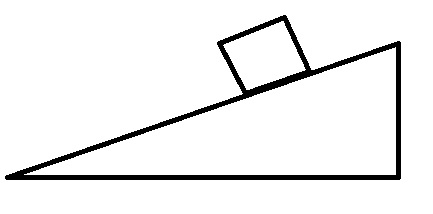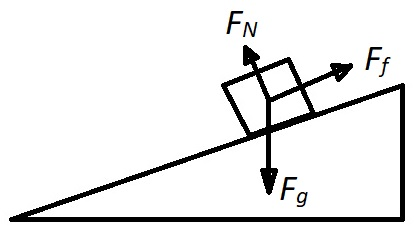Got it
Find the spring constant of a spring if a force of 50 N compresses a spring 5 cm.

Force = -spring constant * extension in meters

5 cm = 0.05 m

50 N = -k * -0.05 m

k = 1,000 N/m

Got it
Coefficient of Sliding Friction

Represented by μs

Dependent on materials of objects in contact

Got it
Find the coefficient of sliding friction for a 10 kg object being pushed at a constant speed with a force of 50 N.

Constant speed, so net force = 0 and applied force = force of friction

Force of sliding friction = coefficient of sliding friction * mass * acceleration of gravity

50 = μs * 10 * 9.8

μs = 0.51

Got it
Types of Friction

Static friction: the force that opposes the motion of a stationary object

Kinetic friction: the force that opposes the motion of an object in motion

Static > kinetic

Got it
Normal Force

The contact force pushing up from a surface onto an object that is in contact with the surface

The force is perpendicular to the surface

Got it
Force

A push or pull by an object onto another object

Vector quantities: have magnitude and direction

Standard unit: Newton (N)

Got it

or choose a specific lesson: See all lessons in this chapter
21 cards in set

## Flashcard Content Overview

The flashcards in this set will give a general overview of forces and gravity. They will describe the types of forces that act on stationary and moving objects and how these can be shown on free-body diagrams. They focus especially on gravity, and how gravity affects objects on Earth.

Front
Back
Force

A push or pull by an object onto another object

Vector quantities: have magnitude and direction

Standard unit: Newton (N)

Normal Force

The contact force pushing up from a surface onto an object that is in contact with the surface

The force is perpendicular to the surface

Types of Friction

Static friction: the force that opposes the motion of a stationary object

Kinetic friction: the force that opposes the motion of an object in motion

Static > kinetic

Find the coefficient of sliding friction for a 10 kg object being pushed at a constant speed with a force of 50 N.

Constant speed, so net force = 0 and applied force = force of friction

Force of sliding friction = coefficient of sliding friction * mass * acceleration of gravity

50 = μs * 10 * 9.8

μs = 0.51

Coefficient of Sliding Friction

Represented by μs

Dependent on materials of objects in contact

Find the spring constant of a spring if a force of 50 N compresses a spring 5 cm.

Force = -spring constant * extension in meters

5 cm = 0.05 m

50 N = -k * -0.05 m

k = 1,000 N/m

Label the forces acting on the object at restAcceleration of Gravity on Earth

Vector quantity represented by g

Is equal to -9.8 m/s2

Negative value because pointing down

Mass

The amount of matter an object has

Weight

The force of gravity on an object

Equal to mass (in kg) times acceleration of gravity (in m/s2)

Standard units = Newtons

Gravity

A pulling force between two objects with mass

Strength dependent on mass of and distance between the objects

A piece of paper and a textbook are pushed off the end of a student's desk. In the absence of air resistance, determine which object hits the floor first.

They both hit the floor at the same time.

Calculate the mass of a person on Earth with a weight of 490 N.

W = m * g

490 N = m * 9.8 m/s2

m = 50 kg = 110 lb

Acceleration Due to Gravity Formula

g = G * M / R2

G is universal gravitational constant

M = mass

R = distance

Calculate the acceleration due to gravity of a planet with the same radius but with 1/5 the mass of Earth.

g = G * M / R2

g = 6.67 * 10-11 * 1/5 * 5.979 * 1024 / (6.376 * 106)2 = 1.96 m/s2

Formula for Newton's Law of Universal Gravitation

F = G (m1 * m2) / r2

G = universal gravitational constant

m1 and m2 = the masses of the two objects

r (or d) = distance between the objects (or the radius of a planet)

Calculate the acceleration due to gravity for objects that are in Earth's exosphere (190,000 km from the surface).

190,000 km = 1.9 * 108 m

g = G * M / R2

g = 6.67 * 10-11 * 5.979 * 1024 / (1.9 * 108 + 6.376 * 106)2 = 0.0103 m/s2

Free Fall

Occurs when an object is moving with gravity as the only force acting on it

Position vs. Time Graph of Free Fall Motion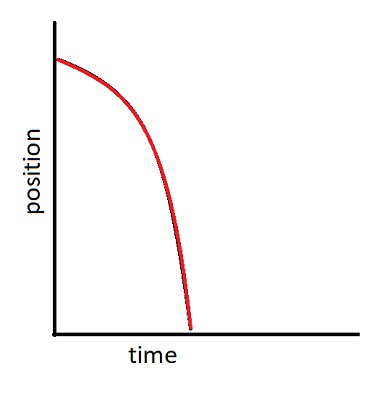Draw a position vs. time graph for an object that starts on the ground, travels up, then free falls back down.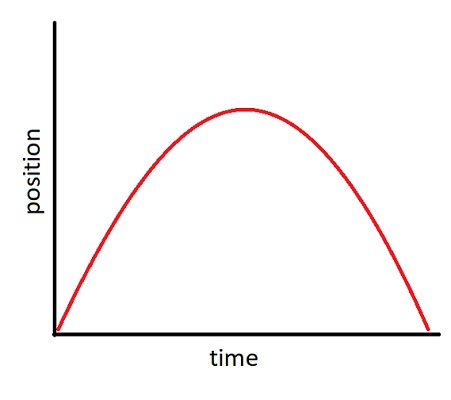Velocity vs. Time Graph of Free Fall Motion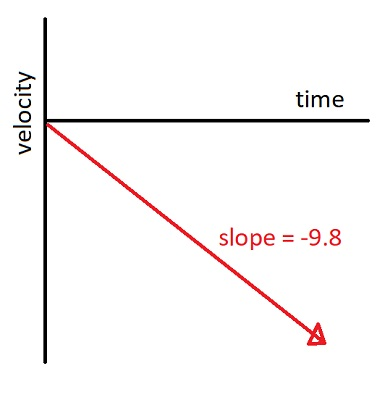To unlock this flashcard set you must be a Study.com Member.